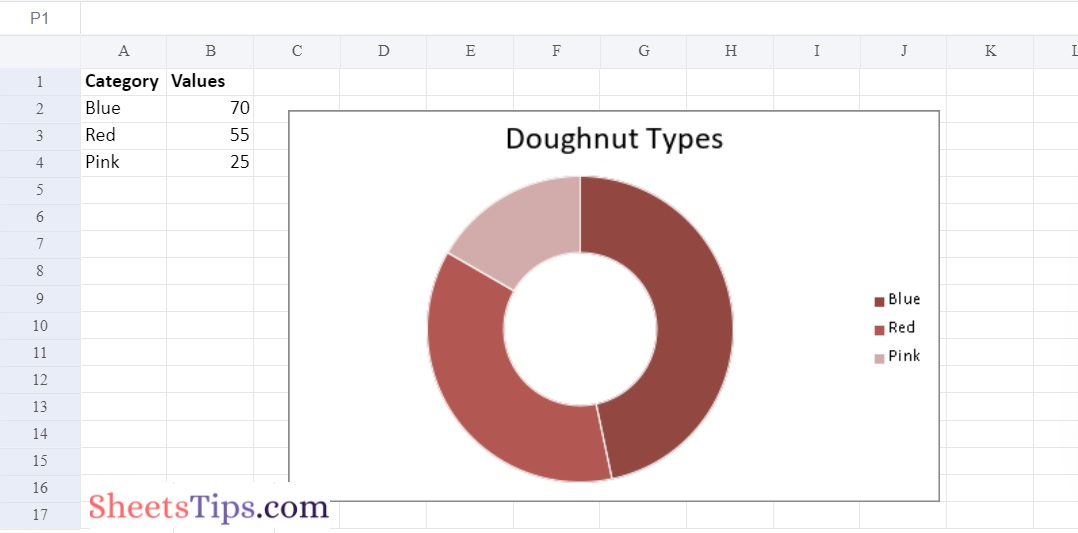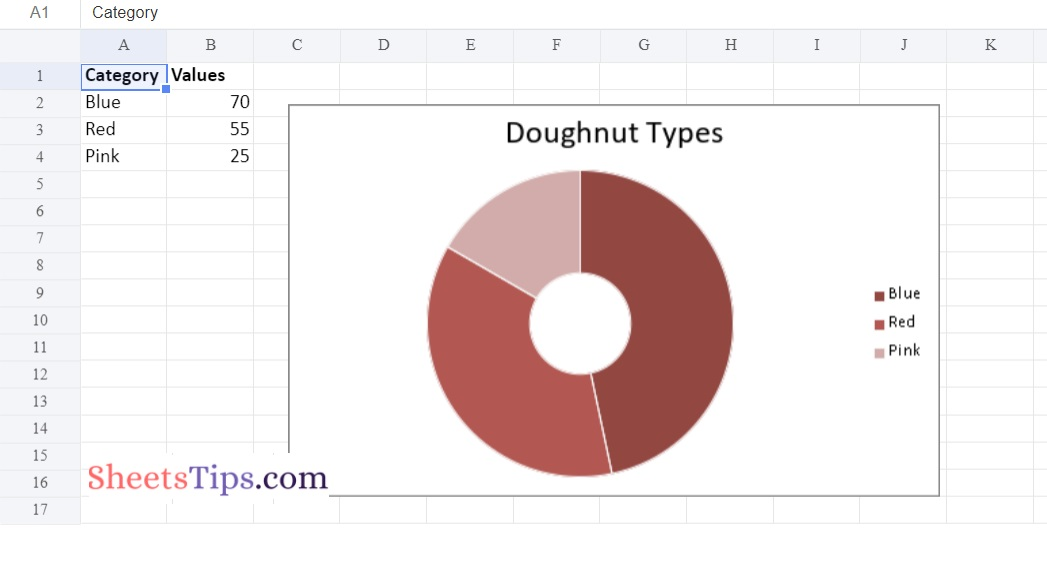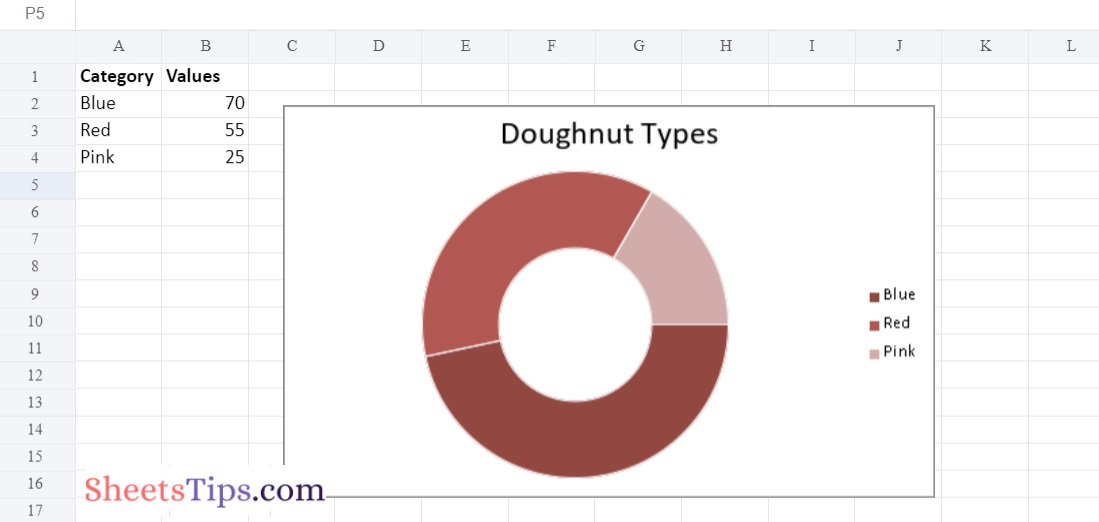# Python Program to Plot Doughnut Charts in Excel sheet using XlsxWriter Module

In this article, we are going to plot the Doughnut Charts in Excel Sheet using XlsxWrite Module in Python.

Charts are made up of a series of one or more data points. The series are made up of references to cell ranges. To plot the charts on an excel sheet, first create a chart object of the desired chart type ( i.e Stock chart, etc.). After generating chart objects, insert data into them before adding them to the sheet object.

Install the xlsxwriter using the below code

`pip install xlsxwriter`

Looking in indexes: https://pypi.org/simple, https://us-python.pkg.dev/colab-wheels/public/simple/
Collecting xlsxwriter
|████████████████████████████████| 149 kB 5.0 MB/s
Installing collected packages: xlsxwriter
Successfully installed xlsxwriter-3.0.3

Recommended Reading On: Python Program to Find the Sum of all Diagonal Elements of a Matrix

## Program to Plot Doughnut Charts in Excel sheet using XlsxWriter Module in Python

Below are the ways to plot the Doughnut Charts in Excel Sheet using XlsxWriter in Python:

### Method #1: Plotting Simple Doughnut Chart

Approach:

• Import the xlsxwriter library using the import function.
• Pass the excel file path to the Workbook() function of xlsxwriter which is the file we want to create and store in a variable.
• Create the new Worksheet using the add_worsheet() function and apply it to the above workbook object and store it in a variable.
• Create a new Format object to format cells in spreadsheets using the add_format() method and apply it to the above workbook object.
• Here, we created a bold format object.
• Add the worksheet data that the charts will use.
• Write a row of heading data starting with ‘A1’ in the bold format using the write_row() function and apply it to the above worksheet object by passing the argument row number, headings list, and format.
• Write the column data that is starting from ‘A2’, ‘B2’ using the write_coulmn() function and pass data as an argument to it.
• Write a column of data starting from ‘A2’, and ‘B2’ respectively.
• Using the add_chart() method, create a chart object that can be added to a worksheet.
• Pass the type of chart as an argument to the add_chart() function and apply it to the above workbook object.
• Using the add series() method, you can add a data series with a pattern to a chart.
• The gap is used to highlight the patterns.
• Configuring the First series.
• Below is the Syntax to define ranges [sheetname, first_row, first_col, last_row, last_col].
• Set the title to the chartObject using the set_title() function by passing the name as value and key as “name” (Here the arguments are object).
• Set the style of the chart using the set_style() function.
• Add a chartObject to the worksheet with the provided offset values in the top-left corner of a chartObject that is anchored to cell C2.
• Close the workbook Object using the close() function.
• The Exit of the Program.

Below is the Implementation:

```# Import the xlsxwriter library using the import function
import xlsxwriter

# Pass the excel file path to the Workbook() function of xlsxwriter
# which is the file we want to create and store it in a variable
workbookObj = xlsxwriter.Workbook('chartDoughnut1.xlsx')

# Create the new Worksheet using the add_worsheet() function and
# apply it to the above workbook object and store it in a variable

# Create a new Format object to format cells in spreadsheets using the add_format() method and apply it to the above workbook object.
# Here, we created a bold format object. .

# Add the worksheet data that the charts will use.
worksheetData = [
['Blue', 'Red', 'Pink'],
[70, 55, 25],
]

# Write a row of heading data starting with 'A1' in bold format using the write_row() function
# and apply on the above worksheet object
# by passing the argument rowNumber,headings list,and format.

# Write the column data that is starting from 'A2','B2' using the write_coulmn() function
# and pass data as argument to it
# Write a column of data starting from
# 'A2', 'B2' respectively .
newWorksheet.write_column('A2', worksheetData)
newWorksheet.write_column('B2', worksheetData)

# Using the add_chart() method, create a chart object that can be added to a worksheet.
# Pass the type of chart as an argument to the add_chart() function
# and apply it to the above workbook object

# Using the add series() method, you can add a data series with a pattern to a chart.
# The gap is used to highlight the patterns.

# Configuring First series.
# Below is the Syntax to define ranges
# [sheetname, first_row, first_col, last_row, last_col].
'name':	 'Doughnut Colour sales data',
'categories': ['Sheet1', 1, 0, 3, 0],
'values':	 ['Sheet1', 1, 1, 3, 1],
})

# Set the title to the chartObject using the set_title() function
# by passing the name as value and key as "name" (Here the arguments are object)
chartObj.set_title ({'name': 'Doughnut Types'})

# Set the style of the chart using the set_style() function
chartObj.set_style(12)

# Add a chartObject to the worksheet with the provided offset values in the top-left corner of a chartObject that is anchored to cell C2.
newWorksheet.insert_chart('C2', chartObj, {'x_offset': 25, 'y_offset': 10})

# Close the workbook Object using the close() function.
workbookObj.close()

```

Output:### Method #2: Plotting a Doughnut chart with rotation of the segments

Set the chart object’s set_rotation() method with a definite angle input to plot a doughnut chart with segment rotation on an excel sheet.

Below is the Implementation:

```# Import the xlsxwriter library using the import function
import xlsxwriter

# Pass the excel file path to the Workbook() function of xlsxwriter
# which is the file we want to create and store it in a variable
workbookObj = xlsxwriter.Workbook('chartDoughnut3.xlsx')

# Create the new Worksheet using the add_worsheet() function and
# apply it to the above workbook object and store it in a variable

# Create a new Format object to format cells in spreadsheets using the add_format() method and apply it to the above workbook object.
# Here, we created a bold format object. .

# Add the worksheet data that the charts will use.
worksheetData = [
['Blue', 'Red', 'Pink'],
[70, 55, 25],
]

# Write a row of heading data starting with 'A1' in bold format using the write_row() function
# and apply on the above worksheet object
# by passing the argument rowNumber,headings list,and format.

# Write the column data that is starting from 'A2','B2' using the write_coulmn() function
# and pass data as argument to it
# Write a column of data starting from
# 'A2', 'B2' respectively .
newWorksheet.write_column('A2', worksheetData)
newWorksheet.write_column('B2', worksheetData)

# Using the add_chart() method, create a chart object that can be added to a worksheet.
# Pass the type of chart as an argument to the add_chart() function
# and apply it to the above workbook object

# Using the add series() method, you can add a data series with a pattern to a chart.
# The gap is used to highlight the patterns.

# Configuring First series.
# Below is the Syntax to define ranges
# [sheetname, first_row, first_col, last_row, last_col].
'name':	 'Doughnut Colour sales data',
'categories': ['Sheet1', 1, 0, 3, 0],
'values':	 ['Sheet1', 1, 1, 3, 1],
})

# Set the title to the chartObject using the set_title() function
# by passing the name as value and key as "name" (Here the arguments are object)
chartObj.set_title ({'name': 'Doughnut Types'})

# Set the style of the chart using the set_style() function
chartObj.set_style(12)
# Change the rotation(angle) of the first segment using ther set_rotation() function
chartObj.set_rotation(90)

# Add a chartObject to the worksheet with the provided offset values in the top-left corner of a chartObject that is anchored to cell C2.
newWorksheet.insert_chart('C2', chartObj, {'x_offset': 25, 'y_offset': 10})

# Close the workbook Object using the close() function.
workbookObj.close()

```

Output:### Method #3: Plotting a Doughnut chart with user defined hole size

Use the set_hole_size() function of the chart object with a specific value(radius) argument to plot a doughnut chart with a user-defined hole size on an excel sheet.

Below is the Implementation:

```# Import the xlsxwriter library using the import function
import xlsxwriter

# Pass the excel file path to the Workbook() function of xlsxwriter
# which is the file we want to create and store it in a variable
workbookObj = xlsxwriter.Workbook('chartDoughnut3.xlsx')

# Create the new Worksheet using the add_worsheet() function and
# apply it to the above workbook object and store it in a variable

# Create a new Format object to format cells in spreadsheets using the add_format() method and apply it to the above workbook object.
# Here, we created a bold format object. .

# Add the worksheet data that the charts will use.
worksheetData = [
['Blue', 'Red', 'Pink'],
[70, 55, 25],
]

# Write a row of heading data starting with 'A1' in bold format using the write_row() function
# and apply on the above worksheet object
# by passing the argument rowNumber,headings list,and format.

# Write the column data that is starting from 'A2','B2' using the write_coulmn() function
# and pass data as argument to it
# Write a column of data starting from
# 'A2', 'B2' respectively .
newWorksheet.write_column('A2', worksheetData)
newWorksheet.write_column('B2', worksheetData)

# Using the add_chart() method, create a chart object that can be added to a worksheet.
# Pass the type of chart as an argument to the add_chart() function
# and apply it to the above workbook object

# Using the add series() method, you can add a data series with a pattern to a chart.
# The gap is used to highlight the patterns.

# Configuring First series.
# Below is the Syntax to define ranges
# [sheetname, first_row, first_col, last_row, last_col].
'name':	 'Doughnut Colour sales data',
'categories': ['Sheet1', 1, 0, 3, 0],
'values':	 ['Sheet1', 1, 1, 3, 1],
})

# Set the title to the chartObject using the set_title() function
# by passing the name as value and key as "name" (Here the arguments are object)
chartObj.set_title ({'name': 'Doughnut Types'})

# Set the style of the chart using the set_style() function
chartObj.set_style(12)

# set the doughnut hole size using the set_hole_size() function by passing the hole size as an argument to it
chartObj.set_hole_size(33)

# Add a chartObject to the worksheet with the provided offset values in the top-left corner of a chartObject that is anchored to cell C2.
newWorksheet.insert_chart('C2', chartObj, {'x_offset': 25, 'y_offset': 10})

# Close the workbook Object using the close() function.
workbookObj.close()

```

Output: